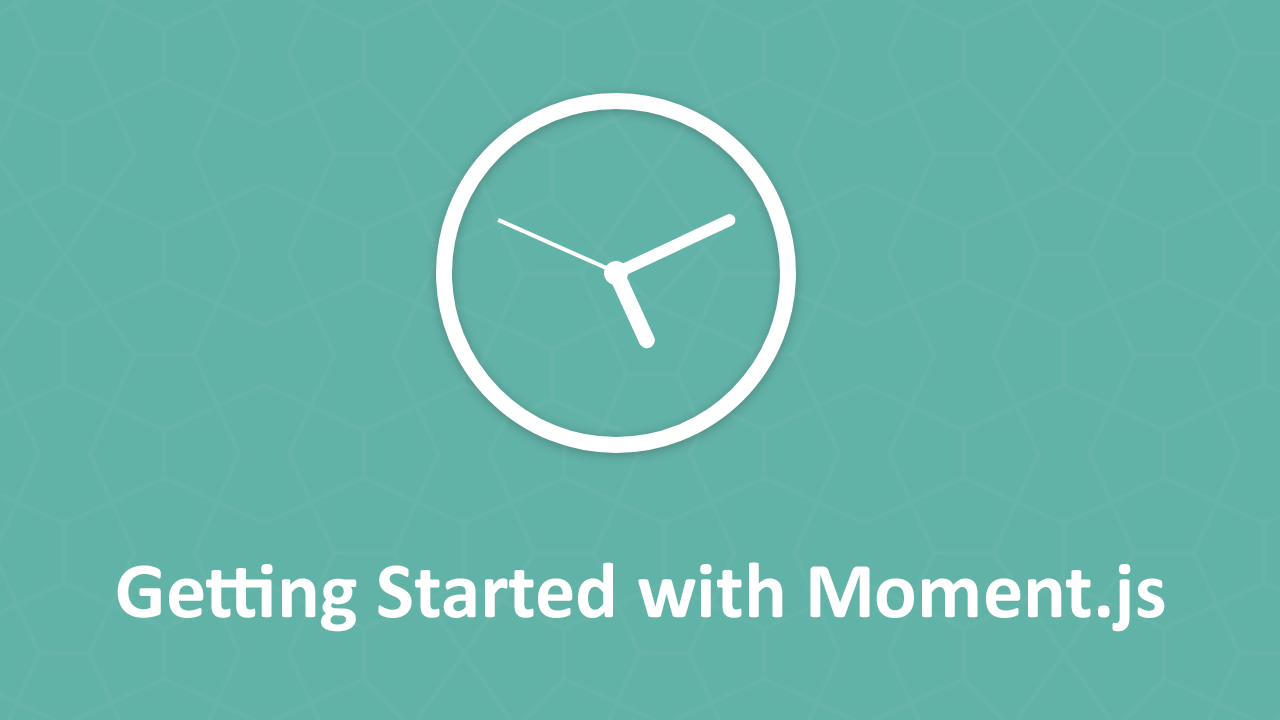# Getting Started with Moment.jsWhile JavaScript provides its own native implementation for handling dates and time, it is known to be a bit difficult and non-intuitive. Because of how common date and time manipulation is, this led to the creation of an open-source JavaScript library called Moment.js that offers an alterative way to validate, parse, and manipulate dates and time.

## Importing Moment.js

Importing Moment.js can be done in a number of ways but we'll just go with the most straight-forward way. Let's use a script tag to import the library:

```	```
<!DOCTYPE html>
<html>
<title>Getting Started with Moment.js</title>
<body>
<script src="https://cdnjs.cloudflare.com/ajax/libs/moment.js/2.22.2/moment.min.js"></script>
</body>
</html>
```
```

Now that we've imported it, let's use it!

## Using Moment.js

Moment.js works by creating a wrapper around the JavaScript Date object. That means to use the library, we must access this wrapper object, which is done by calling `moment()`.

Here's an example:

```	```
<!DOCTYPE html>
<html>
<title>Using Moment.js</title>
<body>
<script src="https://cdnjs.cloudflare.com/ajax/libs/moment.js/2.22.2/moment.min.js"></script>
<script>
let now = moment();
</script>
</body>
</html>
```
```

Using the newly created `now` variable, you can now proceed to do whatever you want with it! Let's take a look at how to format this.

## Formatting a Moment

Now that we have access to the moment object, let's format the date using the `format()` function.

Here's an example of a very common format:

```	```
let now = moment();
console.log(now.format("YYYY MM DD"));
```
```
```	```
2018 09 01
```
```

If you're curious what other letters you may use to format your dates and time, Moment.js supports the ISO 8601 standard.

Here's another example, this time also displaying the time:

```	```
let now = moment();
console.log(now.format("YYYY MM DD - hh:mm:ss a"));
```
```
```	```
2018 09 01 - 12:00:00 am
```
```

## Parsing a Date using Moment.js

You aren't tied to using the current date and time. You can pass in your own date and time and Moment.js will parse it for you. Here's an example of using a custom date by passing in the format of the input:

```	```
let date = moment("2017-09-01", "YYYY-MM-DD");
console.log(date.format("YYYY MM DD - hh:mm:ss a"));
```
```
```	```
2017 09 01 - 12:00:00 am
```
```

## Date Validation

Moment.js offers a really easy way to validate whether or not the date you are working with is valid or not. This is useful for dealing with user input and having the peace of mind that the data inputted is valid. The `isValid()` function returns a boolean indicating whether or not the date is valid.

Here's an example:

```	```
let date1 = moment("2017-09-01");
console.log(date1.isValid());

let date2 = moment("2017-SWAG-01");
console.log(date2.isValid());
```
```
```	```
true
false
```
```

## Date Manipulation

Date manipulation is where Moment.js shines. You can add or subtract any amount of time from a `moment` object to get a new one.

Here are some examples:

```	```
moment().subtract(3, "months") // subtact 3 months from moment
moment().subtract(4, "minutes") // subtact 4 minutes from moment
```
```

Here are all the units of time you can add and subtract:

• milliseconds
• seconds
• minutes
• hours
• days
• weeks
• months
• quarters
• years

## Time Ago and Time Until Date

A very easy way to determine how much time has passed or how much time is left to go relative to another date is to use the `fromNow()` function that returns a readable string representing the time between the two dates.

Let's look at some examples:

```	```
moment("2001-09-01").fromNow(); // 17 years ago
moment("2018-06-01").fromNow(); // 3 months ago
moment("2030-09-01").fromNow(); // in 12 years
```
```

If you don't want the words "in" or "ago", simply pass in `true` as a parameter to the `fromNow()` function:

```	```
moment("2001-09-01").fromNow(); // 17 years
moment("2018-06-01").fromNow(); // 3 months
moment("2030-09-01").fromNow(); // 12 years
```
```

## Conclusion

Moment.js is a powerful open-source library that makes manipulating, parsing, and formatting dates and times extremely simple and straight-forward. We hope this tutorial has been useful to you! Make sure to check out the resoures below so you can become a master at Moment.js!